# Sparse Matrix Reordering

This example shows how reordering the rows and columns of a sparse matrix can influence the speed and storage requirements of a matrix operation.

### Visualizing a Sparse Matrix

A `spy` plot shows the nonzero elements in a matrix.

This `spy` plot shows a sparse symmetric positive definite matrix derived from a portion of the barbell matrix. This matrix describes connections in a graph that resembles a barbell.

```load barbellgraph.mat S = A + speye(size(A)); pct = 100 / numel(A); spy(S) title('A Sparse Symmetric Matrix') nz = nnz(S); xlabel(sprintf('Nonzeros = %d (%.3f%%)',nz,nz*pct));```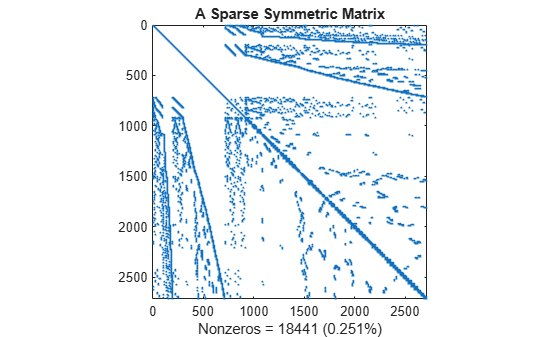Here is a plot of the barbell graph.

```G = graph(S,'omitselfloops'); p = plot(G,'XData',xy(:,1),'YData',xy(:,2),'Marker','.'); axis equal```### Computing the Cholesky Factor

Compute the Cholesky factor `L`, where `S = L*L'`. Notice that `L` contains many more nonzero elements than the unfactored `S`, because the computation of the Cholesky factorization creates fill-in nonzeros. These fill-in values slow down the algorithm and increase storage cost.

```L = chol(S,'lower'); spy(L) title('Cholesky Decomposition of S') nc(1) = nnz(L); xlabel(sprintf('Nonzeros = %d (%.2f%%)',nc(1),nc(1)*pct));```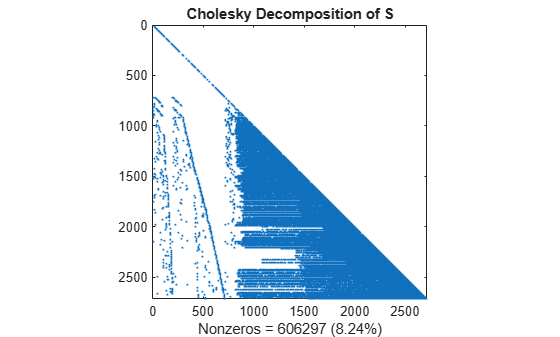### Reordering to Speed Up Calculation

By reordering the rows and columns of a matrix, it is possible to reduce the amount of fill-in that factorization creates, thereby reducing the time and storage cost of subsequent calculations.

Several different reorderings supported by MATLAB® are:

• `colperm`: Column count

• `symrcm`: Reverse Cuthill-McKee

• `amd`: Minimum degree

• `dissect`: Nested dissection

Test the effects of these sparse matrix reorderings on the barbell matrix.

### Column Count Reordering

The `colperm` command uses the column count reordering algorithm to move rows and columns with higher nonzero count towards the end of the matrix.

```q = colperm(S); spy(S(q,q)) title('S(q,q) After Column Count Ordering') nz = nnz(S); xlabel(sprintf('Nonzeros = %d (%.3f%%)',nz,nz*pct));```For this matrix, the column count ordering can barely reduce the time and storage for Cholesky factorization.

```L = chol(S(q,q),'lower'); spy(L) title('chol(S(q,q)) After Column Count Ordering') nc(2) = nnz(L); xlabel(sprintf('Nonzeros = %d (%.2f%%)',nc(2),nc(2)*pct));```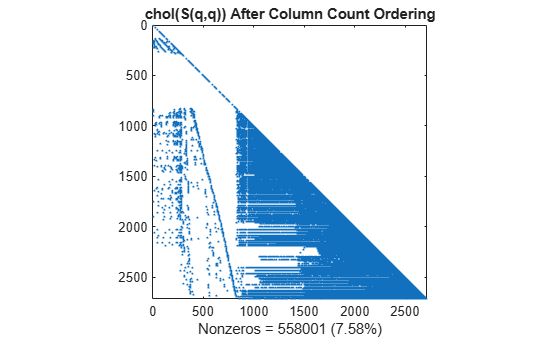### Reverse Cuthill-McKee Reordering

The `symrcm` command uses the reverse Cuthill-McKee reordering algorithm to move all nonzero elements closer to the diagonal, reducing the bandwidth of the original matrix.

```d = symrcm(S); spy(S(d,d)) title('S(d,d) After Cuthill-McKee Ordering') nz = nnz(S); xlabel(sprintf('Nonzeros = %d (%.3f%%)',nz,nz*pct));```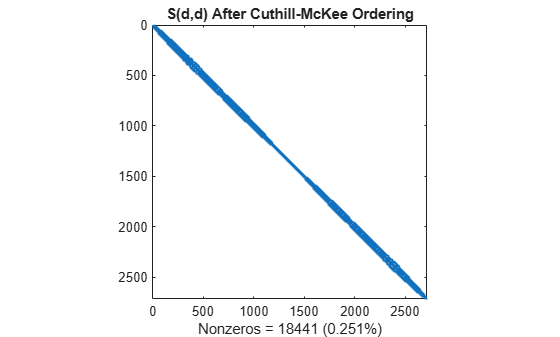The fill-in produced by Cholesky factorization is confined to the band, so factorizing the reordered matrix takes less time and less storage.

```L = chol(S(d,d),'lower'); spy(L) title('chol(S(d,d)) After Cuthill-McKee Ordering') nc(3) = nnz(L); xlabel(sprintf('Nonzeros = %d (%.2f%%)', nc(3),nc(3)*pct));```### Minimum Degree Reordering

The `amd` command uses an approximate minimum degree algorithm (a powerful graph-theoretic technique) to produce large blocks of zeros in the matrix.

```r = amd(S); spy(S(r,r)) title('S(r,r) After Minimum Degree Ordering') nz = nnz(S); xlabel(sprintf('Nonzeros = %d (%.3f%%)',nz,nz*pct));```The Cholesky factorization preserves the blocks of zeros produced by the minimum degree algorithm. This structure can significantly reduce time and storage costs.

```L = chol(S(r,r),'lower'); spy(L) title('chol(S(r,r)) After Minimum Degree Ordering') nc(4) = nnz(L); xlabel(sprintf('Nonzeros = %d (%.2f%%)',nc(4),nc(4)*pct));```### Nested Dissection Permutation

The `dissect` function uses graph-theoretic techniques to produce fill-reducing orderings. The algorithm treats the matrix as the adjacency matrix of a graph, coarsens the graph by collapsing vertices and edges, reorders the smaller graph, and then uses refinement steps to uncoarsen the small graph and produce a reordering of the original graph. The result is a powerful algorithm that frequently produces the least amount of fill-in compared to the other reordering algorithms.

```p = dissect(S); spy(S(p,p)) title('S(p,p) After Nested Dissection Ordering') nz = nnz(S); xlabel(sprintf('Nonzeros = %d (%.3f%%)',nz,nz*pct));```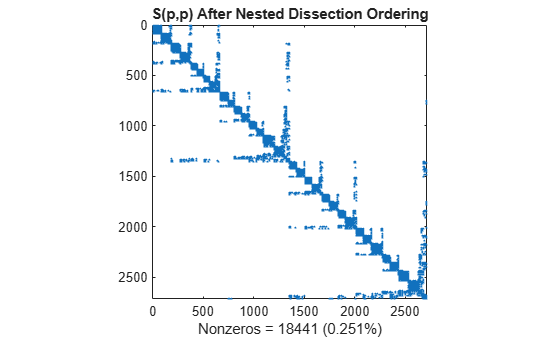Similar to the minimum degree ordering, the Cholesky factorization of the nested dissection ordering mostly preserves the nonzero structure of `S(d,d)` below the main diagonal.

```L = chol(S(p,p),'lower'); spy(L) title('chol(S(p,p)) After Nested Dissection Ordering') nc(5) = nnz(L); xlabel(sprintf('Nonzeros = %d (%.2f%%)',nc(5),nc(5)*pct));```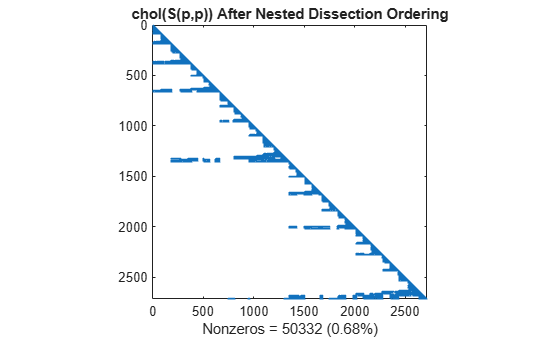### Summarizing Results

This bar chart summarizes the effects of reordering the matrix before performing the Cholesky factorization. While the Cholesky factorization of the original matrix had about 8% of its elements as nonzeros, using `dissect` or `symamd` reduces that density to less than 1%.

```labels = {'Original','Column Count','Cuthill-McKee',... 'Min Degree','Nested Dissection'}; bar(nc*pct) title('Nonzeros After Cholesky Factorization') ylabel('Percent'); ax = gca; ax.XTickLabel = labels; ax.XTickLabelRotation = -45;```